# Popsicle Party

Franklin School is having a Popsicle party for its nine hundred fifty students. Each student will get two Popsicles. Popsicles come in boxes that have one dozen Popsicles. There are twenty-five boxes in a carton. The principal is sure that the number of Popsicles in the cartons bought will go equally into the number of Popsicles needed for the students. The secretary says that there will be some Popsicles left over to share with the teachers. How many complete cartons of Popsicles does Franklin School need? Explain if the principal or the secretary is correct and why.

Assessment

## Plan

#### Formal Mathematical Language and Symbolic Notation

A student may independently select a printed number line, number chart, ten frames, graph paper, etc. as they work on a task.

### Suggested materials

Engagement Image:

Teachers may project the image below to launch this task for their students, define nouns, promote discussion, access prior knowledge, and inspire engagement and problem solving.

## Solutions tabs

### Expert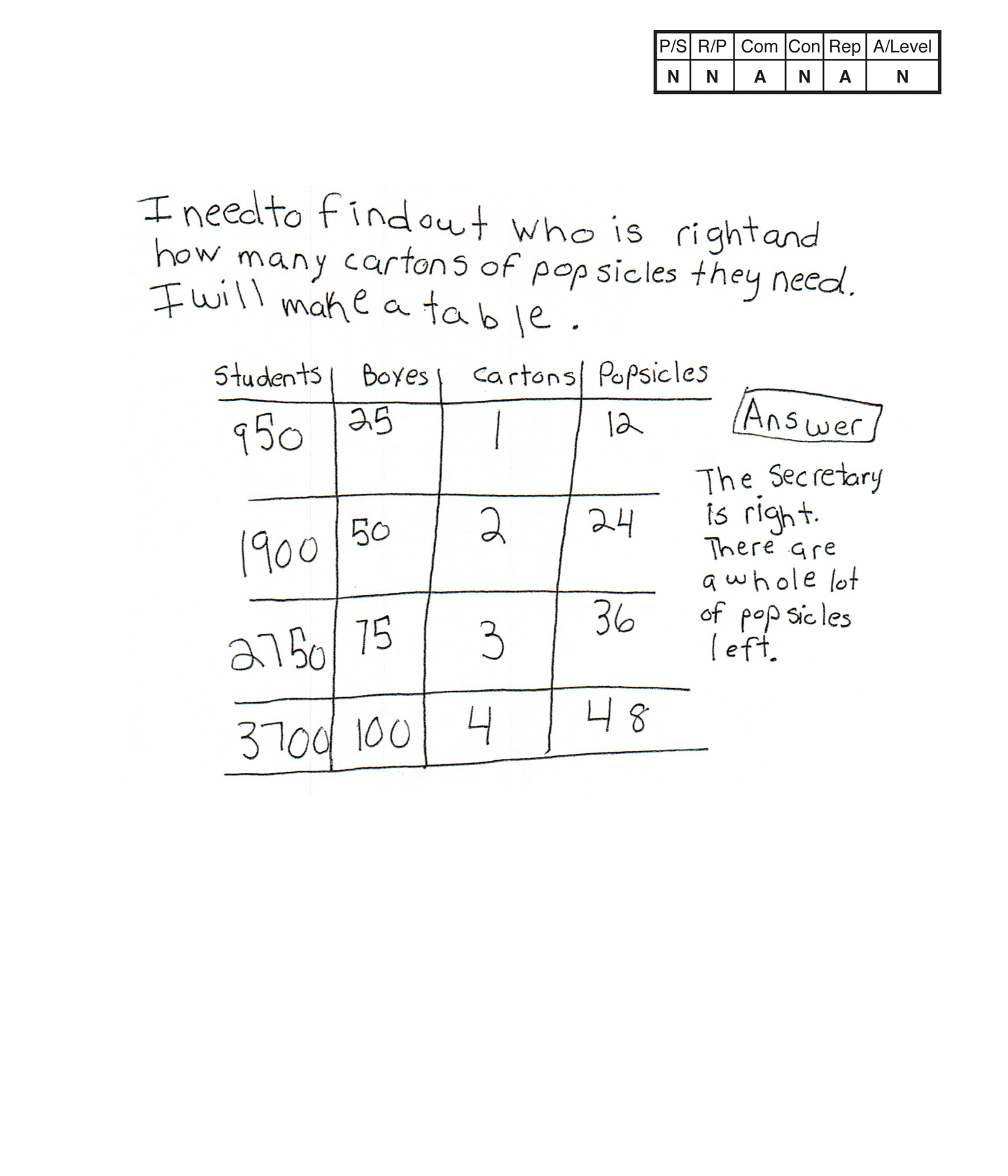This student does not meet the standard.

### Scoring Rationale

#### Novice

The student's strategy of making a table including repeated addition of 950 students to 3,700 students, repeated addition of 25 boxes to 100 boxes, and repeated addition of 12 Popsicles to 48 Popsicles does not work to solve the task. The student's answer, "The secretary is right. There are a whole lot of popsicles left," is not correct as it is based on incorrect problem solving.

#### Novice

The student does not demonstrate correct reasoning of the underlying concepts of the task. It appears that the student is adding 950 students twice for 1,900 students, adding on again with a computational error for 2,750 students, and then adding 950 again for 3,700 students. The student is also adding on 25 boxes for a total of 100 boxes, and 12 Popsicles for a total of 48 Popsicles. The student does not determine that the task only involves 1,900 students and that 25 boxes equals 300 Popsicles so seven cartons are needed to have enough Popsicles for 950 students to each have two Popsicles.

#### Apprentice

The student correctly uses the mathematical term table.

#### Novice

The student solves the task and does not make a mathematically relevant connection.

#### Apprentice

The student's table is appropriate but not accurate. The third row of the student's first column should state 2,850 to represent the student's thinking. The rest of the data does represent the student's incorrect reasoning.

#### Novice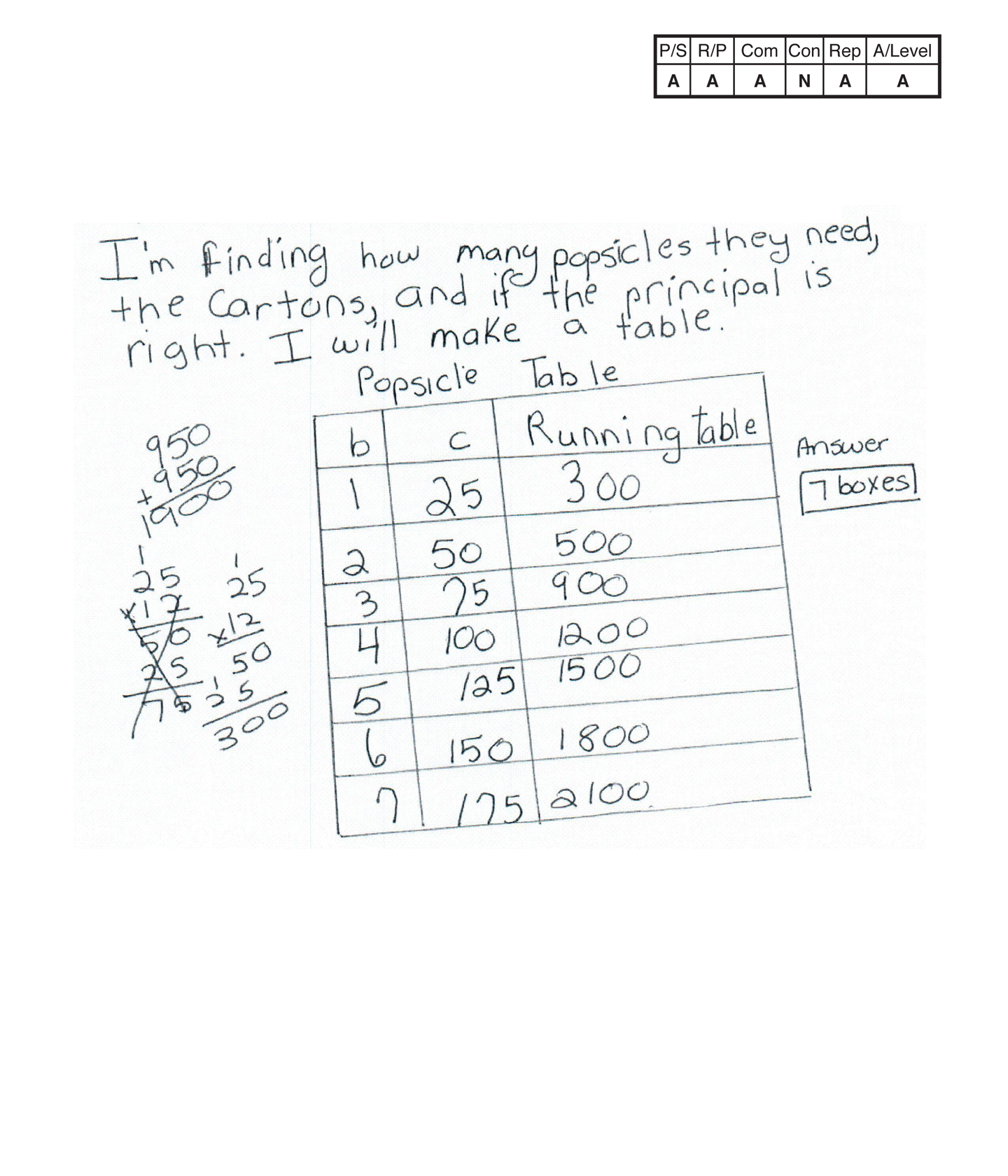This student does not meet the standard.

### Scoring Rationale

#### Apprentice

The student's strategy of making a table to show the number of boxes and cartons of Popsicles needed for 950 students to each have two Popsicles would work to solve this task. The student switches the data for boxes and cartons and makes a calculation error for the running total in the second row. The student is able to state a correct answer, "7 boxes," but does not explain if the principal or the secretary is right and why.

#### Apprentice

The student demonstrates understanding of the underlying concepts of doubling the students for 1,900 Popsicles, finding 300 Popsicles for a dozen boxes and continuing to seven boxes. It appears that the student switches the boxes and cartons data and has a calculation error. The student does not show understanding to determine if the principal or the secretary is right and why.

#### Apprentice

The student correctly uses the mathematical term table. The correct label for the third column should be running total and not running table.

#### Novice

The student solves the task and does not make a mathematical connection.

#### Apprentice

The student's table is appropriate but not accurate. The first column should be labeled cartons, the second column should be labeled boxes, and the third column should be labeled running total. The student does not provide a key to define "b" and "c."

#### Apprentice

Note:

The overall achievement level for this piece of student work falls under Exemplars exception to the rule category. If a student has all Apprentice scores or above, but a Novice in "Connections," the student may still receive an achievement level score of Apprentice. To learn more about Exemplars scoring, please refer to the section of your dashboard called "Tools for Success" and click on the link for "Using the Assessment Rubric."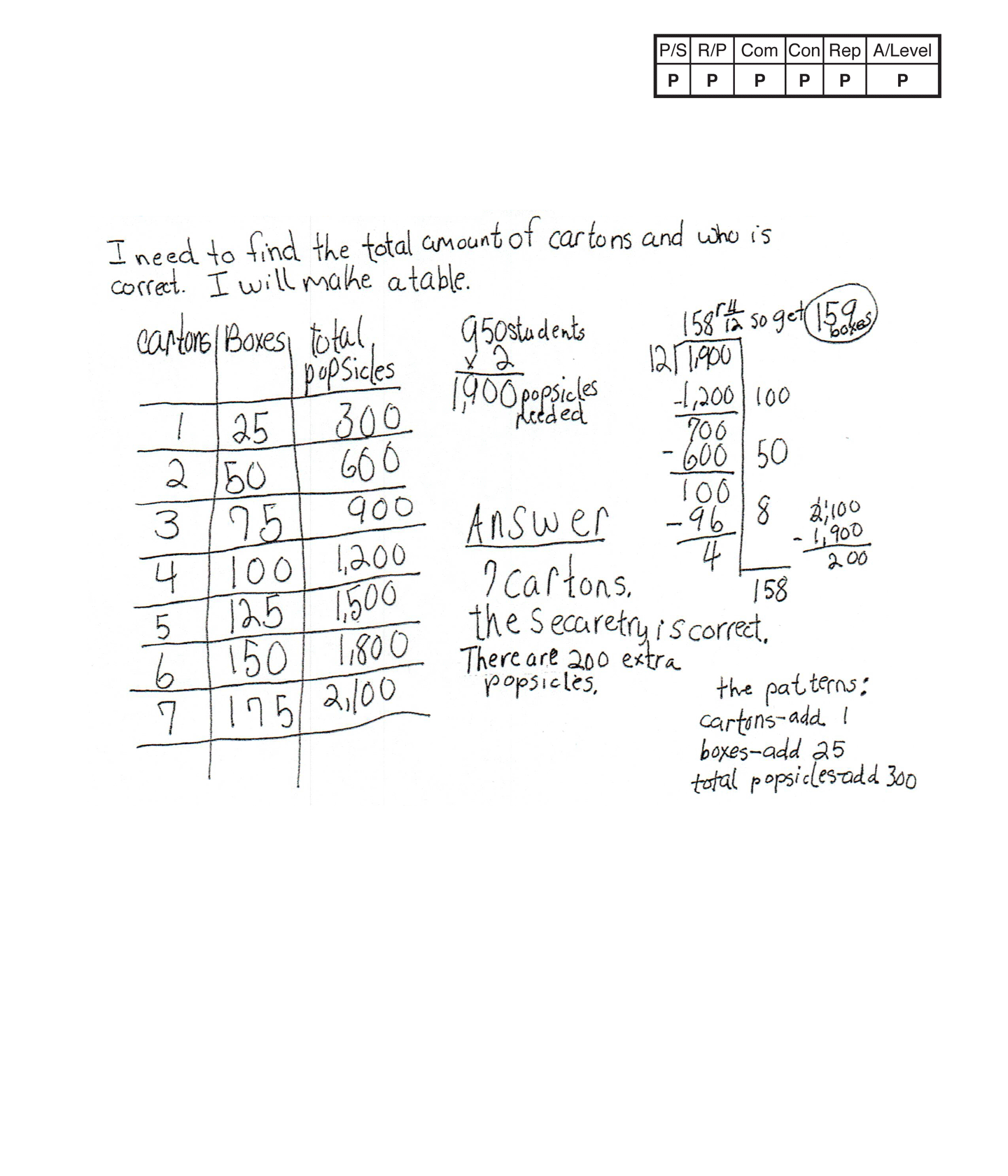This student meets the standard.

### Scoring Rationale

#### Practitioner

The student's strategy of making a table to determine the number of cartons, boxes, and total Popsicles needed for 950 students to each have two Popsicles works to solve part of the task. The student also explains why the secretary is correct to address the second part of the task. The student's answers, "7 cartons," and "the secaretry is correct. There are 200 extra popsicles," is correct.

#### Practitioner

The student demonstrates understanding of the underlying concepts of doubling the students for 1,900 Popsicles, finding a total of 300 Popsicles for each carton of 25 boxes per carton, and continuing for seven cartons. The student also uses division to determine how many boxes of Popsicles are needed. The student shows correct reasoning in determining that the secretary is correct because there are 200 remaining Popsicles after each student receives two Popsicles.

#### Practitioner

The student correctly uses the mathematical terms total, amount, table, patterns.

#### Practitioner

The student makes the mathematically relevant observation of finding patterns in their table. The student states, "The patterns: cartons—add 1, boxes—add 25, total popsicles—add 300."

#### Practitioner

The student's table is appropriate and accurate. All necessary labels are provided and the entered data is correct.

#### Practitioner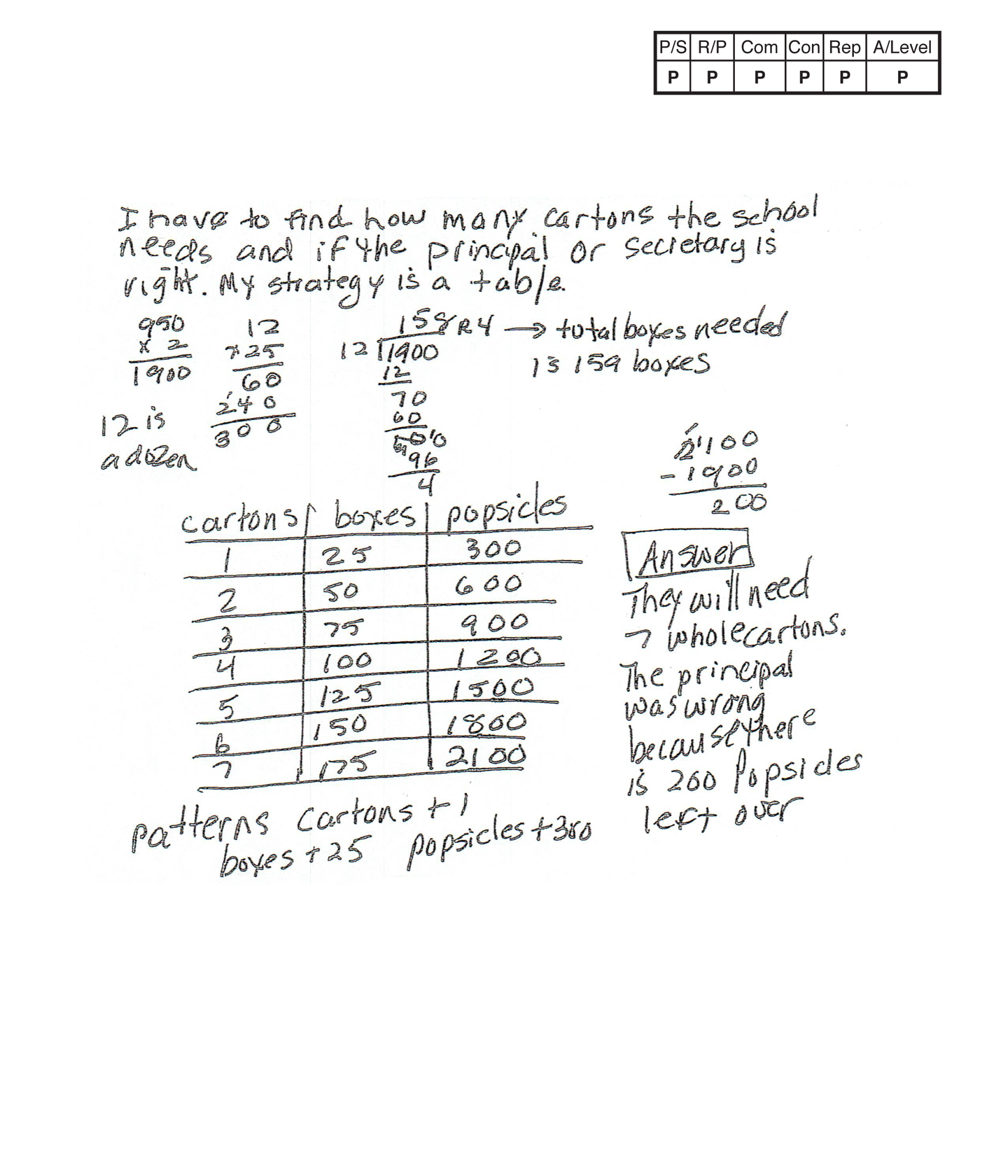,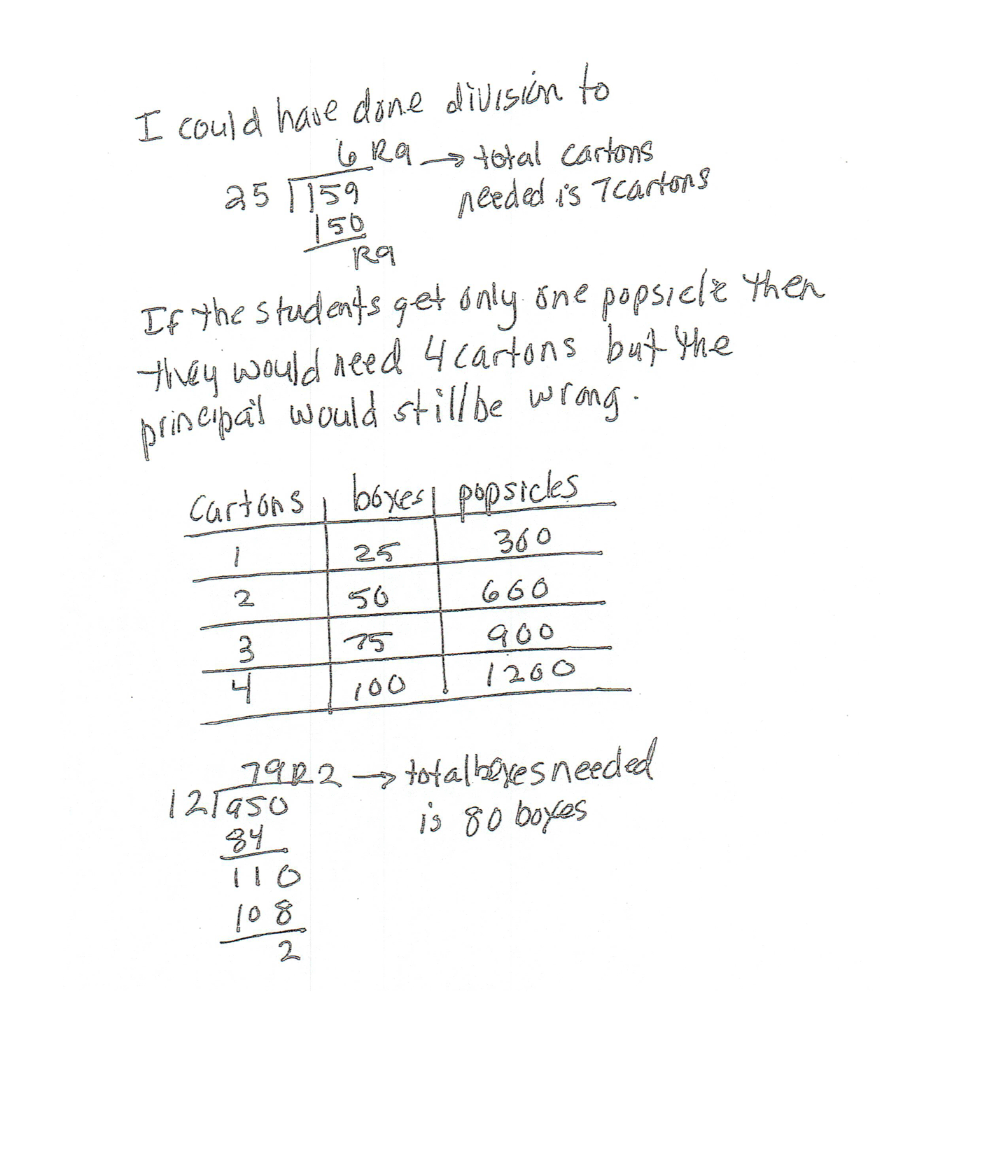This student meets the standard.

### Scoring Rationale

#### Practitioner

The student's strategy of making a table to show the number of cartons, boxes, and Popsicles needed for 950 students to each have two Popsicles works to solve the first part of the task. The student also explains why the principal is wrong to answer the second part of the task. The student's answer, "They will need 7 whole cartons. The principal was wrong because there is 200 Popsicles left over," is correct.

#### Practitioner

The student demonstrates understanding of the underlying concepts of doubling the students for 1,900 Popsicles, finding 300 Popsicles for a dozen boxes and continuing to determine that seven cartons of Popsicles are needed. The student also shows correct reasoning in determining that the principal is wrong because there are 200 Popsicles remaining after each student receives two Popsicles.

#### Practitioner

The student correctly uses the mathematical term dozen from the task. The student also correctly uses the terms table, total, whole, patterns, division.

#### Practitioner

The student uses a second strategy of division to determine that seven cartons are needed for 950 students. The student states, "I could have done division to," and "total cartons needed is 7 cartons." The student recreates the task and finds a new answer. "If the students get only one popsicle then they would need 4 cartons but the principal would still be wrong." The student uses a table and division to support this new task.

#### Practitioner

The student's tables are appropriate and accurate. All labels are provided and the data is correct. Only one table needs to be appropriate and accurate to earn a Practitioner level.

#### Practitioner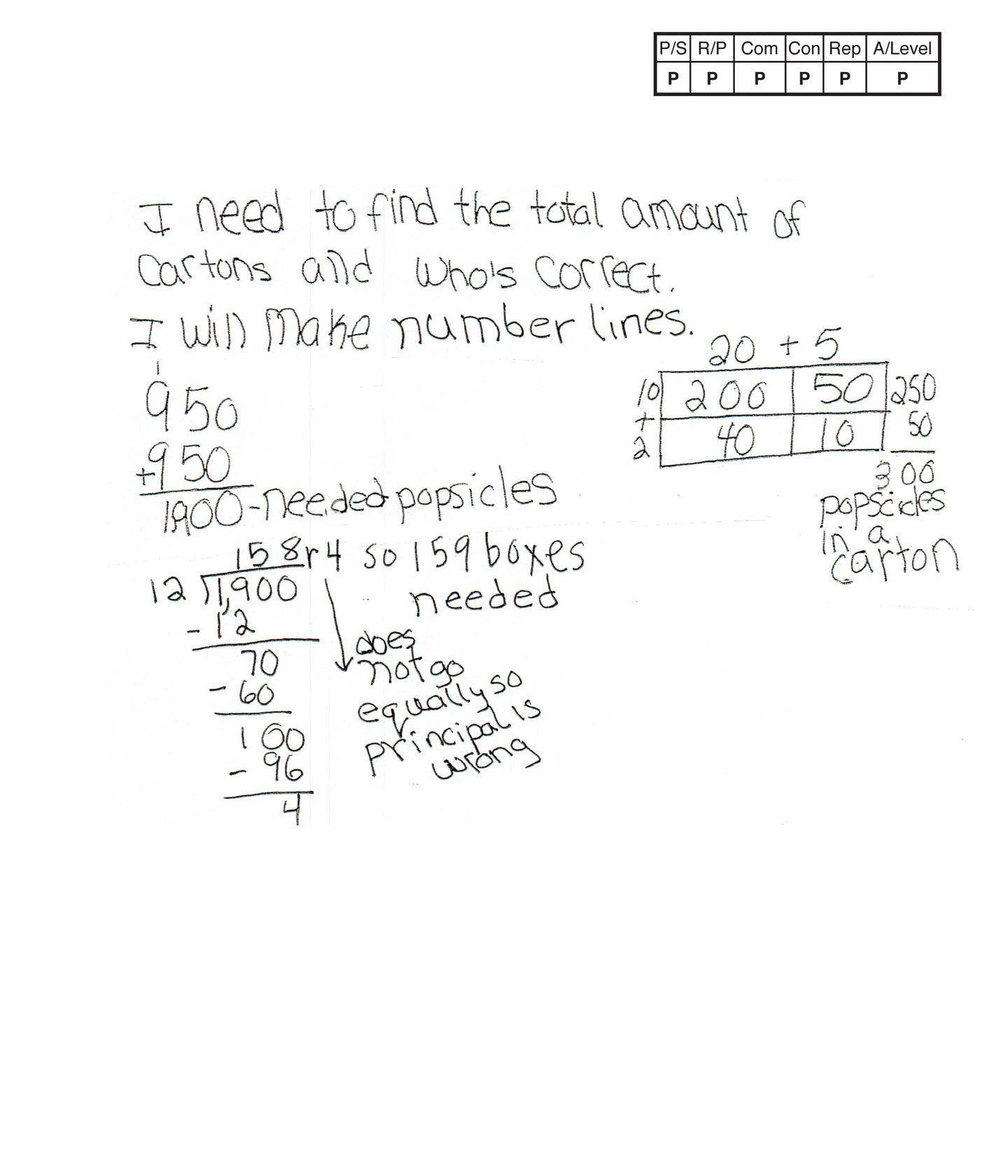,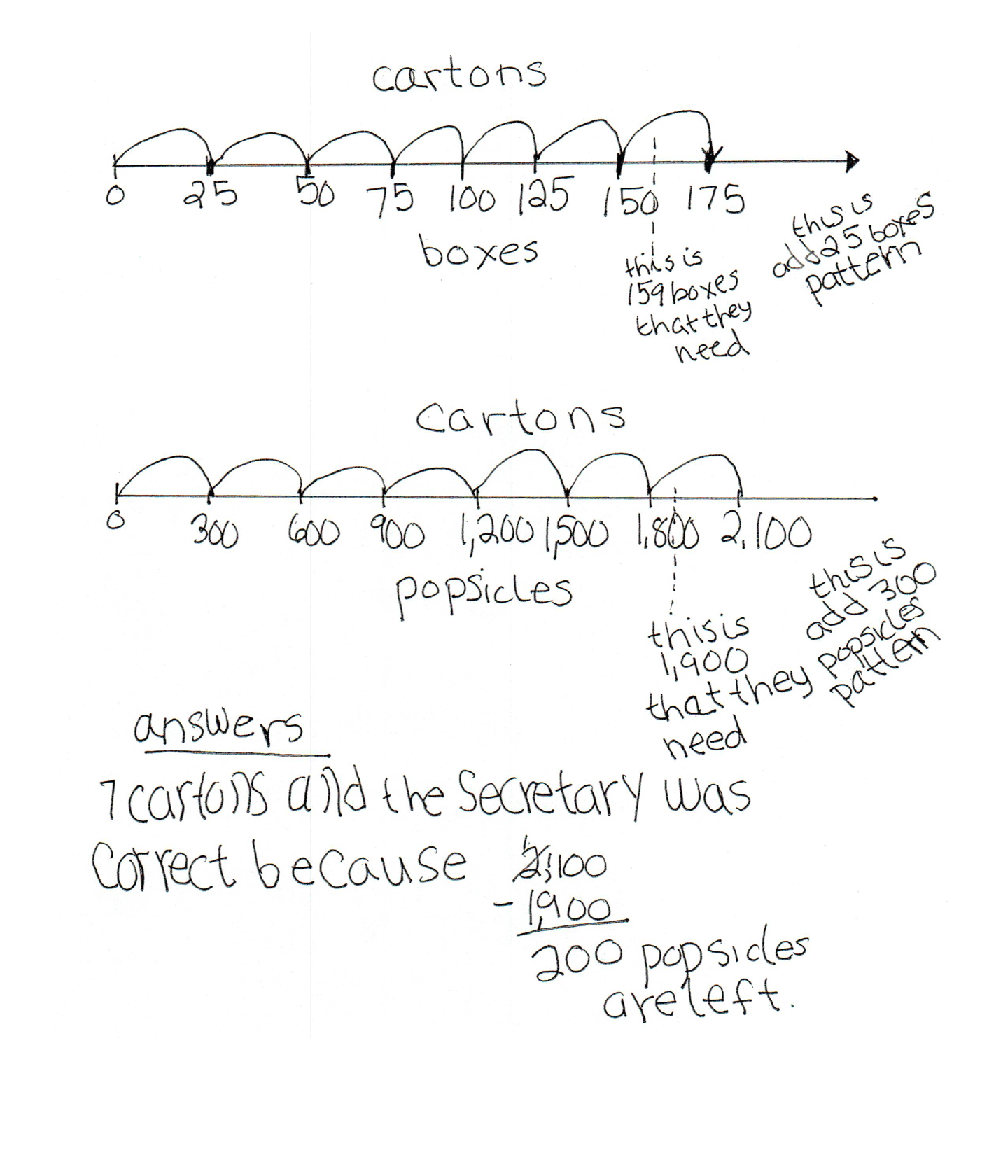This student meets the standard.

### Scoring Rationale

#### Practitioner

The student's strategy of making number lines to determine the number of cartons, boxes, and total Popsicles needed for 950 students to each have two Popsicles works to solve the first part of the task. The student also explains why the secretary is correct to address the second part of the task. The student's answers, "7 cartons and the secretary was correct because 2,100 - 1,900 = 200 popsicles are left," is correct.

#### Practitioner

The student demonstrates understanding of the underlying concepts of doubling the students for 1,900 Popsicles, finding a total of 300 Popsicles for each carton of 25 boxes per carton, and that 159 boxes of Popsicles are needed. The student shows correct reasoning in determining that the secretary is correct because there are 200 remaining Popsicles after each student receives two Popsicles.

#### Practitioner

The student correctly uses the mathematical term equally from the task. The student also correctly uses the terms total, amount, number lines, pattern.

#### Practitioner

The student makes the mathematically relevant observation of finding patterns in their table. The student states, "this is add 25 boxes pattern," and "this is add 300 popsicles pattern."

#### Practitioner

The student's number lines are appropriate and accurate. All necessary labels are provided, the intervals are spaced correctly and the "jumps" for number of cartons are correct.

#### Practitioner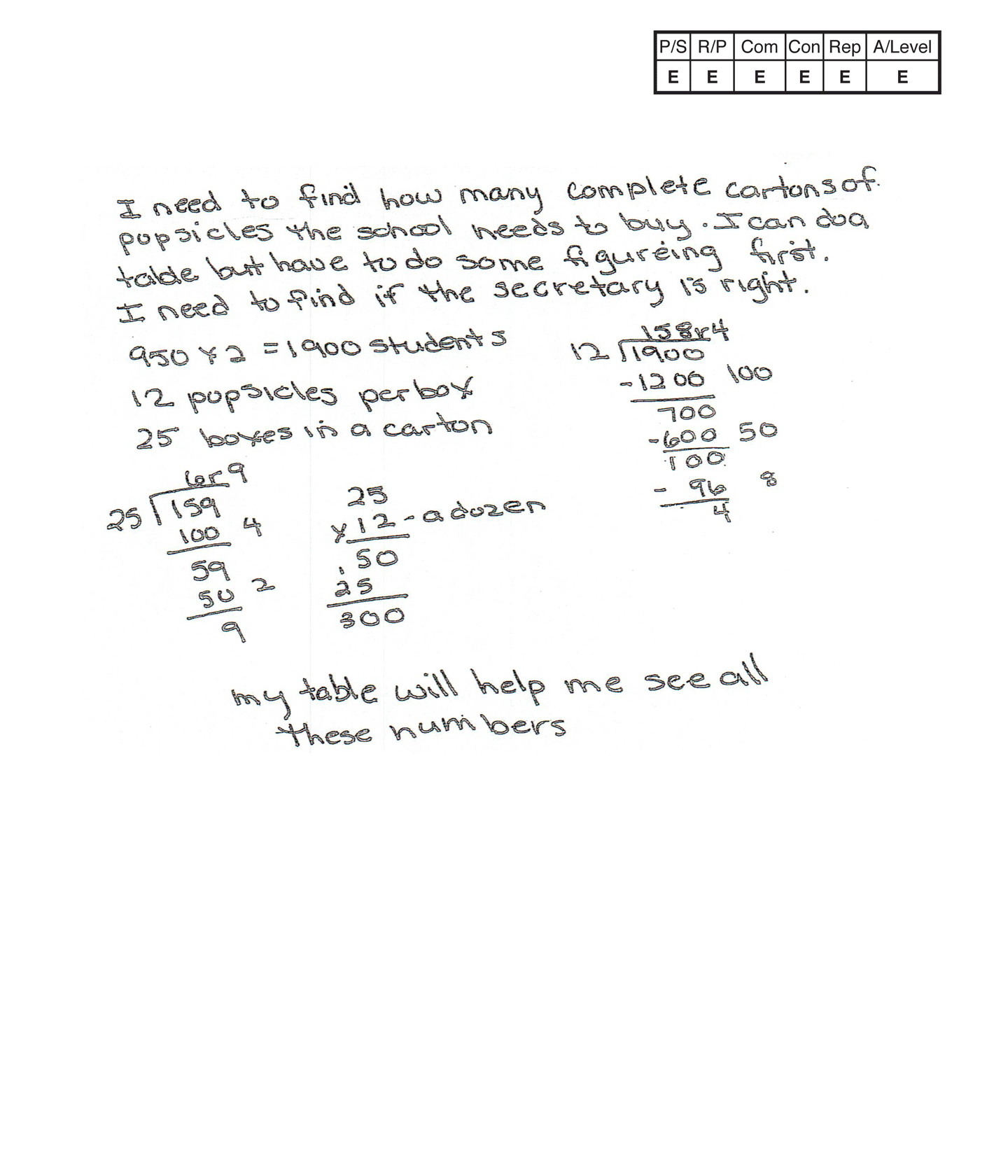,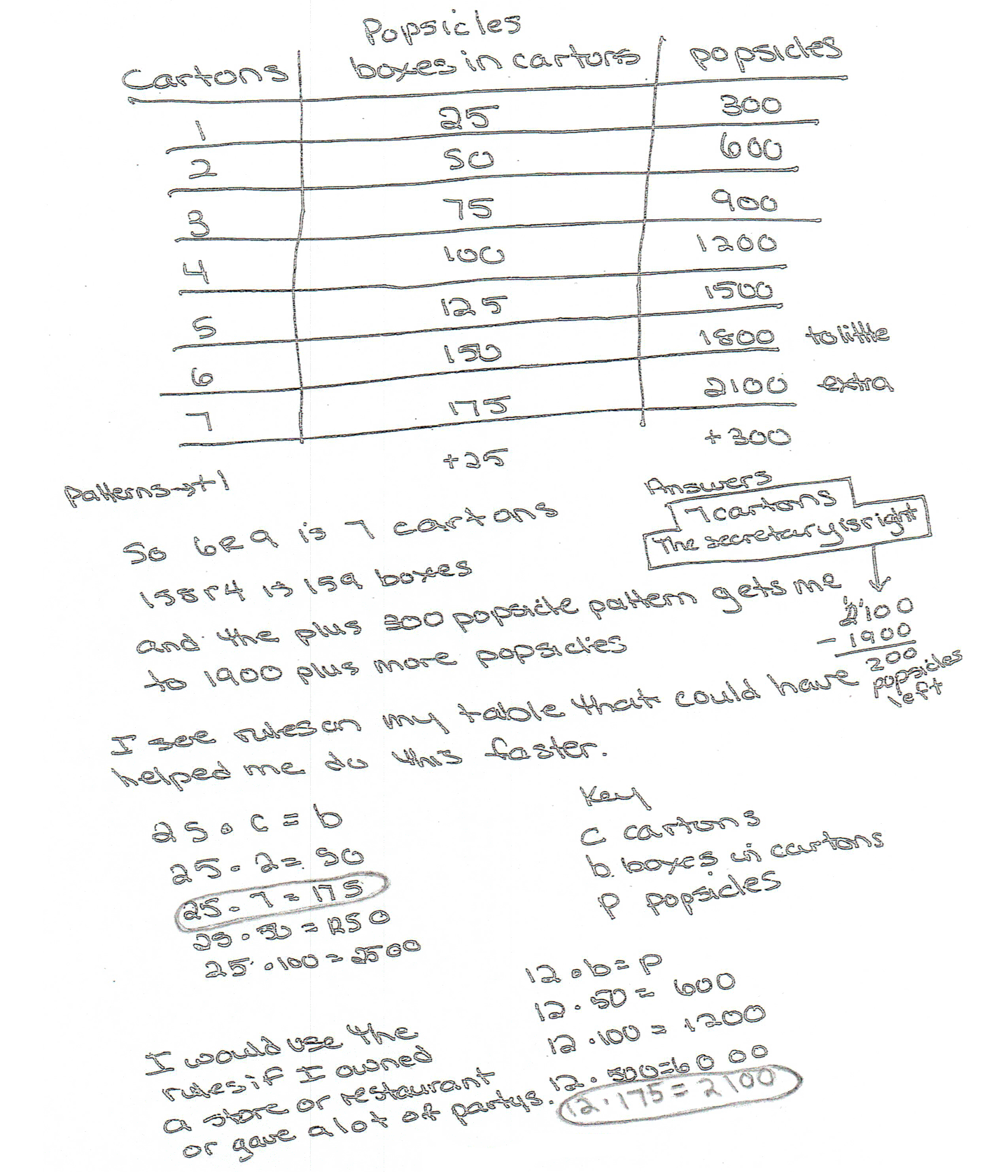This student exceeds the standard.

### Scoring Rationale

#### Expert

The student's strategy of using computation to find the number of Popsicles needed for 950 students to have two each, the number of Popsicles in 25 boxes, how many dozen Popsicles are in 1,900 Popsicles, and the number of cartons needed as well as using a table to display this computation works to solve the first part of the task. The student also determines that the secretary was right because there are "200 popsicles left," to address the second part of the task. The student's answer, "7 cartons," and "The secretary is right," is correct. The student also uses an alternate strategy of a generalized rule to find the number of boxes and Popsicles needed for the students.

#### Expert

The student demonstrates understanding of the underlying concepts of doubling the students for 1,900 Popsicles, finding 300 Popsicles for a dozen boxes and continuing to determine that seven cartons of Popsicles are needed. The student applies an understanding of division to determine seven cartons and 159 boxes are needed. The student also shows correct reasoning in determining that the secretary is correct because there are 200 remaining Popsicles. The student supports their decisions by stating, "I see rules on my table what could have helped me do this faster."

#### Expert

The student correctly uses the mathematical term dozen from the task. The student also correctly uses the terms table, numbers, per, pattern, more, rules, key. The student correctly uses the mathematical notation 25 • c = b," and "12 • b = p," with all variables defined in their key.

#### Expert

The student makes the Practitioner mathematical observations, "patterns + 1" (for cartons), "+ 25" (for boxes), and "+ 300” (for Popsicles). The student then makes the Expert connection by generalizing two rules that can be used to find the number of boxes for any number of cartons, "25 • c = b", and the number of Popsicles for any number of boxes, "12 • b = p." The student uses the rules to find the total boxes for two, seven, 50 and 100 cartons, and the Popsicles for 50, 100, 500, and 175 boxes.

#### Expert

The student's table is appropriate and accurate. All labels are provided and the data is correct. The student uses their table to determine two rules. "I see rules on my table that could have helped me do this faster."